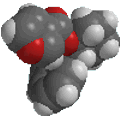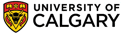Useful ConceptsCombustion Data

This is probably best illustrated by working through some examples. The problems are each slightly different, but the basic strategy is similar, you need to work out how much of each element is present and the simplest ratio between them.

A 0.30g of an unknown organic compound X gave 0.733g of carbon dioxide and 0.30g of water in a combustion analysis. Determine the empirical formula.

How many moles of CO2 and H2O are generated ?
CO2 : 0.733g / 44.009 g/mol = 16.66 mmol. and for H2O: 0.30g / 18.015g/mol = 16.66 mmol.

Remembering that the equation for a combustion reaction tells us that we will get one molecule of CO2 for each atom of C and one molecule of H2O for every 2 H atoms, then the ratio of C:H for X is 1: 2 corresponding to 0.30 g of X containing 16.66 mmol of C and 33.3 mmol of H.

To check the total composition, this should be converted to a %.
% C = (16.66 mmol * 12.011) / 0.30g = 66.6 %
% H = (33.3 mmol * 1.008) / 0.30g = 11.3 %
This does not add up to 100%, therefore, assume that the balance is oxygen, O = 22.1 % which corresponds to 0.221 * 0.30g = 0.066g or 4.14 mmol.

Now one needs to determine the stoichiometry in X. To do thisI suggest you make a little table, writing in the contributing atoms and the amount present (mmol) in the sample, then the empirical ratio can be found by dividing by the smallest.

 Atoms C H O mmol 16.66 33.3 4.14 / smallest 3.999 7.998 1.000 emp. ratio 4 8 1

THEREFORE, the empirical formula is C4H8O

In order to determine the molecular formula, it is necessary to know the molecular weight.
(RESIST the temptation to round the numbers by more than a few hundredths, otherwise you will probably get the wrong answer !)

B An unknown organic compound Z gave 8.8 g of carbon dioxide and 4.5 g of water in a combustion analysis for C and H. Z was found to have a molecular weight = 58.124 g/mol. Determine the molecular formula.

How many moles of CO2 and H2O are generated ?
CO2 : 8.8 g / 44.009 g/mol = 0.20 mol. and for H2O: 4.50g / 18.015g/mol = 0.25 mol.

Remembering that the equation for a combustion reaction tells us that we will get one molecule of CO2 for each atom of C and one molecule of H2O for every 2 H atoms, then the ratio of C:H for Z is 0.20 : 0.50 indicating an empirical ratio C2H5.

Alternatively, to do this, you can make a little table, writing in the contributing atoms and the amount present (mmol) in the sample, then the empirical ratio can be found by dividing by the smallest.

 Atoms C H mol 0.20 2 x 0.25 / smallest 1.00 2.5 emp. ratio 2 5

THEREFORE, the empirical ratio is C2H5

This empirical unit has mass: = (2 * 12.011) + (5 * 1.008) = 29.062

How many of these units are in the molecule ? 58.124 / 29.062 = 2

THEREFORE, the molecular formula is C4H10

(RESIST the temptation to round the numbers by more than a few hundredths, otherwise you will probably get the wrong answer !)

C An unknown organic compound Y gave 8.8 g of carbon dioxide and 4.5 g of water in a combustion analysis for C and H. Z was found to have a molecular weight = 74.123 g/mol. Determine the molecular formula.

How many moles of CO2 and H2O are generated ?
CO2 : 8.8 g / 44.009 g/mol = 0.20 mol. and for H2O: 4.50g / 18.015g/mol = 0.25 mol.

Remembering that the equation for a combustion reaction tells us that we will get one molecule of CO2 for each atom of C and one molecule of H2O for every 2 H atoms, then the ratio of C:H for Y is 0.20 : 0.50 indicating an empirical ratio C2H5.

Alternatively, to do this, you can make a little table, writing in the contributing atoms and the amount present (mmol) in the sample, then the empirical ratio can be found by dividing by the smallest.

 Atoms C H mol 0.20 2 x 0.25 / smallest 1.00 2.5 emp. ratio 2 5

THEREFORE, the empirical ratio is C2H5

This empirical unit has mass: = (2 * 12.011) + (5 * 1.008) = 29.062

How many of these units are in the molecule ? 74.239 / 29.062 = 2.55 (!)

Since this is not an integer, another element must be present....

Need some trial and error now.... the minimum number of those units that can be present is 2...... 2 x 29.062 = 58.124
Which implies we are missing 74.123 - 58.124 = 15.999
Now check the periodic table to see what is most likely to be missing.... in this case it suggests one oxygen atom...
(Note this can be tricky if you need to consider multiple atoms etc. However, if this is normally the case, then other information would have to be provided)

THEREFORE, the molecular formula is C4H10O

(RESIST the temptation to round the numbers by more than a few hundredths, otherwise you will probably get the wrong answer !)

Elemental Analysis Data

Essentially this is similar to problem A above (but simpler !). Three examples are presented.

A. An unknown organic compound X gave the following elemental analysis data : C = 88.16%, H = 11.84%. What is the empirical formula of X ?

First thing to do is check the total %. In this case it is 100%, therefore, we have a hydrocarbon.

If you then imagine that you are considering a 100g sample then by dividing by the atomic weights you get the moles of each atom type present. Dividing through by the smallest then gives the empirical ratio and after scaling to give the simplest integer ratios, the empirical formula is obtained.

 Atoms C H % 88.16 11.84 / A.W 7.34 11.75 / smallest 1 1.60 emp. ratio 5 8

THEREFORE, the empirical formula is C5H8

(note.... the scaling is often the tough part, here we have an initial ratio of 1 : 1.60, so you need to find the first multiple of 1.6 that is a whole number (or very close to it). Avoid the temptation to round the first two decimal places.

B. An unknown organic compound Y gave the following elemental analysis data : C = 58.8%, H = 9.8%. What is the empirical formula of Y?

First thing to do is check the total %. It is not 100%, therefore, since we are not given any other information, we should assume that the balance is oxygen, O = 31.4 % (see footnote).
To solve the rest of the problem, we suggest you make a little table, writing in the contributing atoms and the amount present (%) in the sample. If you then imagine that you are considering a 100g sample then by dividing by the atomic weights you get the moles of each atom type present. Dividing through by the smallest then gives the empirical ratio and after scaling to give the simplest integer ratios, the empirical formula is obtained.

 Atoms C H O % 58.8 9.8 31.4 / A.W 4.9 9.8 1.96 / smallest 2.5 5.0 1.0 emp. ratio 5 10 2

THEREFORE, the empirical formula is C5H10O2

C. An unknown organic compound Z gave the following elemental analysis data : C = 66.63%, H = 11.18% and molecular weight = 72.107 g/mol. What is the molecular formula of Z?

First thing to do is check the total %. It is not 100%, therefore, since we are not given any other information, we should assume that the balance is oxygen, O = 22.19 %. (see footnote).

In this case, we know the molecular weight, so the problem is simplified. We can work out the mass in one mole that corresponds to each element by multiplying the MW by the % of the element.
Then dividing by the atomic weights you get the moles of each atom type present.

 Atoms C H O % 66.63 11.18 22.19 % * MW 48.04 8.06 16.00 / A.W 3.996 7.996 1.000 ratio 4 8 1

THEREFORE, the molecular formula is C4H8O

Footnote : Since elemental analysis data is derived from combustion analysis, it involves the combustion of the sample in a stream of oxygen. This means one can not measure the amount of oxygen present in the sample and hence it can only be assumed based on the missing %. Note that routine or standard elemental analysis is for the elements C, H and N. As a cross check, before commiting to assuming that the missing % is oxygen, check to see if any other data suggests any other element is present (e.g. Br or Cl). If no other data is provided (or available), then all you can do is assume that it is oxygen. If N was found it the elemental analysis, it would be reported as a % N.© Dr. Ian Hunt, Department of Chemistry